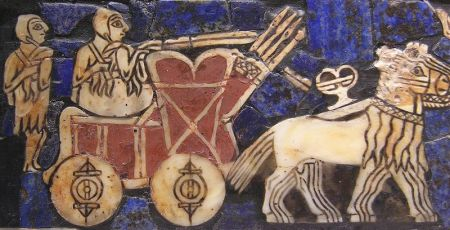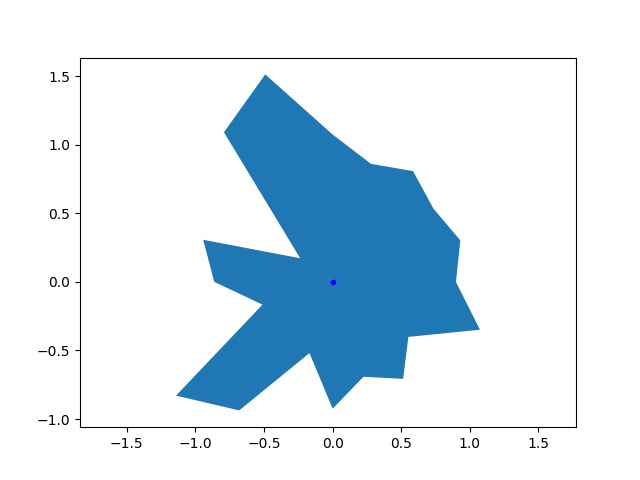# Reinventing the Wheel: Discovering the Optimal Rolling Shape with PyTorchIt is thought that the wheel was invented more than five thousand years ago, yet modern machine learning tools were only developed in the past few decades. We have all wondered about this paradox at some point. How could ancient Sumerian wheel builders have calculated the ideal shape without modern computers and software? We may never know. Luckily, with today's technology, it is relatively easy to rediscover the wheel's optimal shape. I'll show you how I did it.The goal of this goofy project was to find the optimal shape of a wheel, which is probably a circle. I parameterized the wheel as a twenty sided polygon with a variable radius for each vertex, $$r_i$$. The performance of the wheel was based on the final speed achieved by an accelerating imaginary car, $$v_f$$. The wheel was driven with a constant torque, $$\tau$$, and no slipping. I used PyTorch to simulate the effect of a wheel and calculate the gradients in the final speed with respect to the radii, $$\frac{dv_f}{dr_i}$$. I used these gradients to update the radii using gradient descent. Above, you can see the shape of an initially randomly generated wheel the wheel evolving, along with the changing objecive function.

I represented the wheel with a relatively simple model. In each time step, I updated the speed of the car based on the force from the wheel, then rotated the wheel based on the speed. The lowest vertex was touching the ground and was fixed in space. The wheel generated a force on the car $$\tau/r_i$$, where $$r_i$$ is the radius of the vertex. A component of this force, shown in the diagram below, accelerated the car and increased its speed.Next, I think it would be fun to try surfaces that aren't flat. If I used that rolling square surface, would the optimal shape be a square?